Question

# Fig. 1 shows a circuit of four capacitors connected to a battery with a potential difference...

Fig. 1 shows a circuit of four capacitors connected to a battery with a potential difference ?_0. Capacitor 4 is filled with a dielectric with 0 dielectric constant ? = 4. The capacitance value of the capacitors is equal ?1 =?2 = ?3 = ?. The capacitance of capacitor 4 is also equal to ? / 2 when in the middle of
their plates there is no dielectric. a) Calculate the free charge stored in the capacitor 4. Its
answer must be in thermal of ? and ?. b) Calculate the charge and the potential difference in capacitors ?1, ?2 and ?3.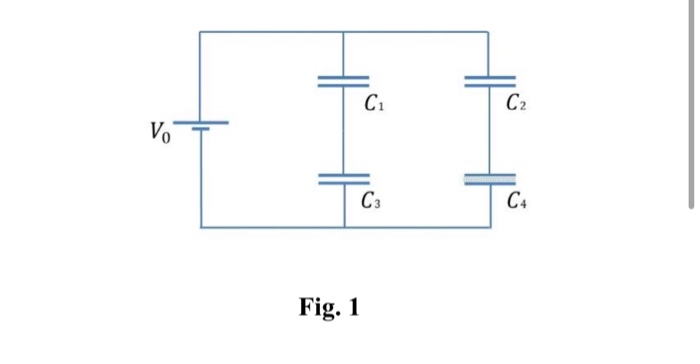C1 C2 Vo C3 C4 Fig. 1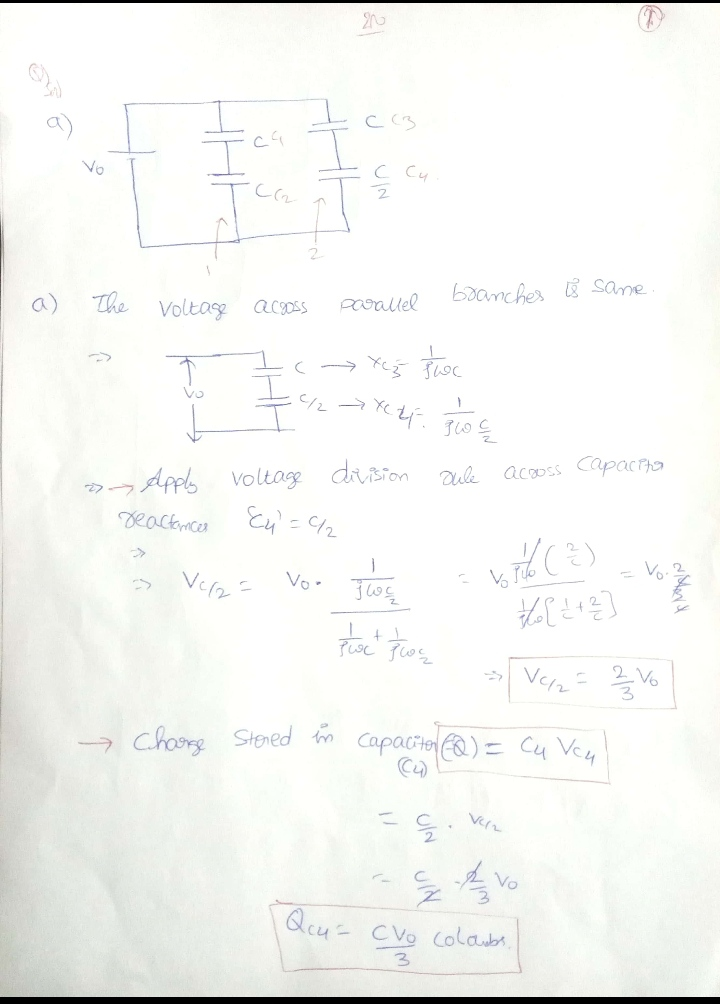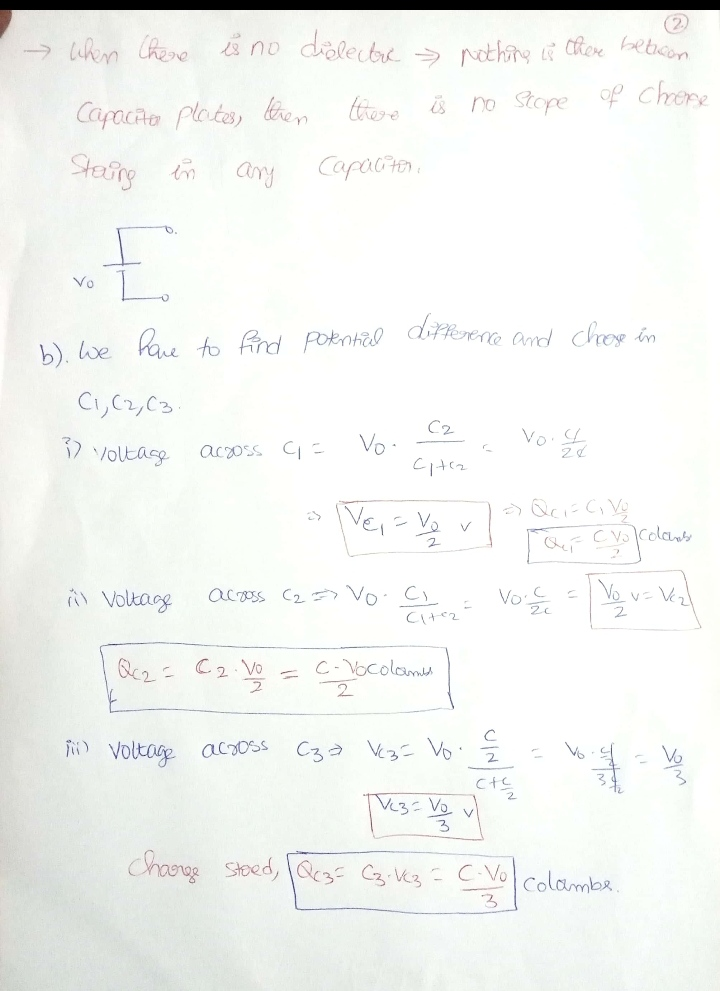#### Earn Coins

Coins can be redeemed for fabulous gifts.

Similar Homework Help Questions
• ### Two capacitors are connected to each other and the battery is removed. The voltage across each...

Two capacitors are connected to each other and the battery is removed. The voltage across each capacitor is 5.5 volts. V = V1 = V2 = 5.5 V. The capacitance of C1 = 1.0 x 10 ^-6 F and the capacitance of C2= 2.0 x 10 ^-6 F. What is the charge on each capacitor? If the gap between the plates of C1 if filled with paraffin (dielectric constant K=2.2) what is the new charge on each capacitor and what...

• ### The figure shows a circuit with an ideal battery and set of capacitors. The battery has...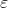The figure shows a circuit with an ideal battery and set of capacitors. The battery has an emf =12 V. The capacitors have capacitances C1 = C2 = C3 = C4 = 6 nF. (a) What is the equivalent capacitance of the entire network of capacitors? (b) What is the electrical energy stored in the capacitor with capacitance C2? We were unable to transcribe this imageProblem 6 C. The figure shows a circuit with an ideal battery and set of...

• ### The circuit in the figure below shows four capacitors connected to a battery. The switch S...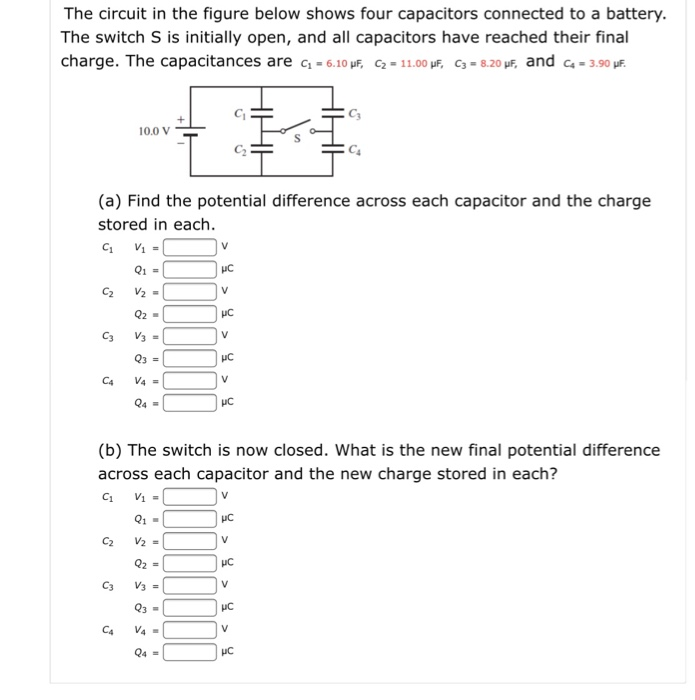The circuit in the figure below shows four capacitors connected to a battery. The switch S is initially open, and all capacitors have reached their final charge. The capacitances are ci-6.10 F, C2. 11.00,F, C3.8.20,f, and ca. 3.90pE 00V (a) Find the potential difference across each capacitor and the charge stored in each HC (b) The switch is now closed. What is the new final potential difference across each capacitor and the new charge stored in each? HC IC Q4-...

• ### Four capacitors are arranged in the circuit shown in the figure. The capacitors have the values...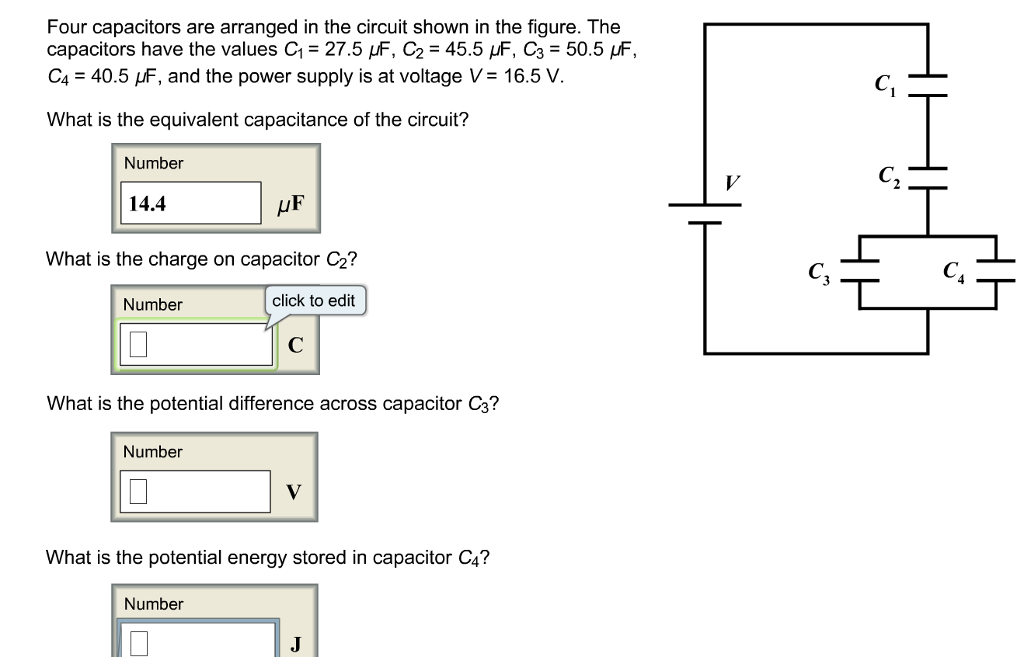Four capacitors are arranged in the circuit shown in the figure. The capacitors have the values C1 = 27.5 μF, C2 = 45.5 μF, C3 = 50.5 μF, C4 = 40.5 μF, and the power supply is at voltage V = 16.5 V. What is the equivalent capacitance of the circuit? Four capacitors are arranged in the circuit shown in the figure. The capacitors have the values C1-27.5 μF, C2 = 45.5 μF, C3 = 50.5 F, C.-40.5 and the...

• ### The circuit in the figure below shows four capacitors connected to a battery. The switch s...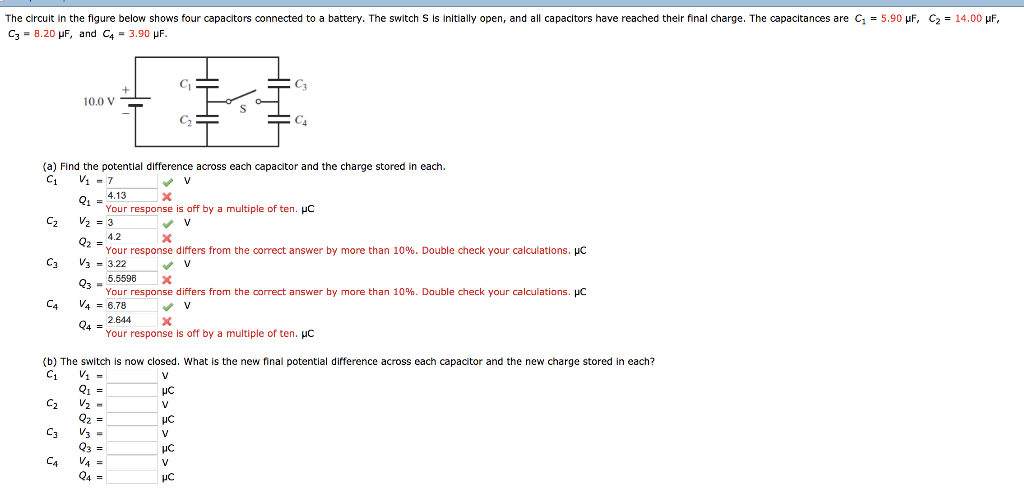The circuit in the figure below shows four capacitors connected to a battery. The switch s is initially open, and all capacitors have reached their final charge. The capacitances are C1 = 5.90 μF, C3 = 8.20 μF, and C4 = 3.90 μF C2 = 14.00 μF 10.0 V (a) Find the potential difference across each capacitor and the charge stored in each. 4.13 Your response is off by a multiple of ten. HC 4.2 Your response differs from the...

• ### In the circuit shown on the right, with all capacitors fully charged:  the potential difference...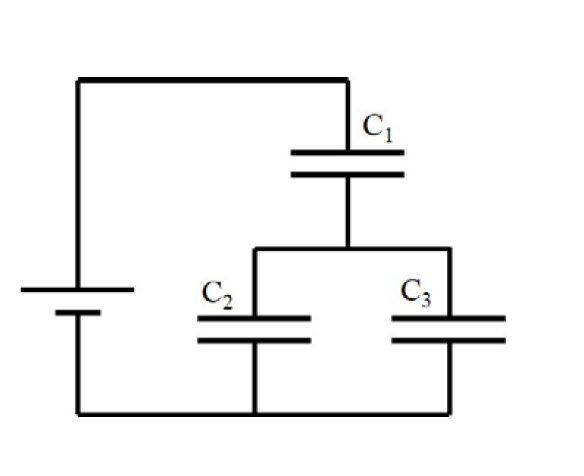In the circuit shown on the right, with all capacitors fully charged:  the potential difference across C1 is equal to 5.0 V  the charge stored on C1 is equal to 2.5 μC  the charge stored on C2 is equal to 1.2 μC  the capacitance of C3 is equal to 0.65 μF Calculate the total equivalent capacitance of the circuit. 3 2

• ### A circuit is constructed with five capacitors and a battery as shown. The values for the...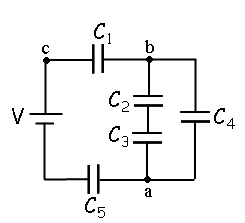A circuit is constructed with five capacitors and a battery as shown. The values for the capacitors are: C1 = C5 = 4.2 μF, C2 = 1 μF, C3 = 5.8 μF, and C4 = 4.4 μF. The battery voltage is V = 12 V. 1) What is Cab, the equivalent capacitance between points a and b?μF 2) What is Cac, the equivalent capacitance between points a and c? 3) What is Q5, the charge on capacitor C5?μC 4) What...

• ### 20 of 22 > Four capacitors are arranged in the circuit shown in the figure. The...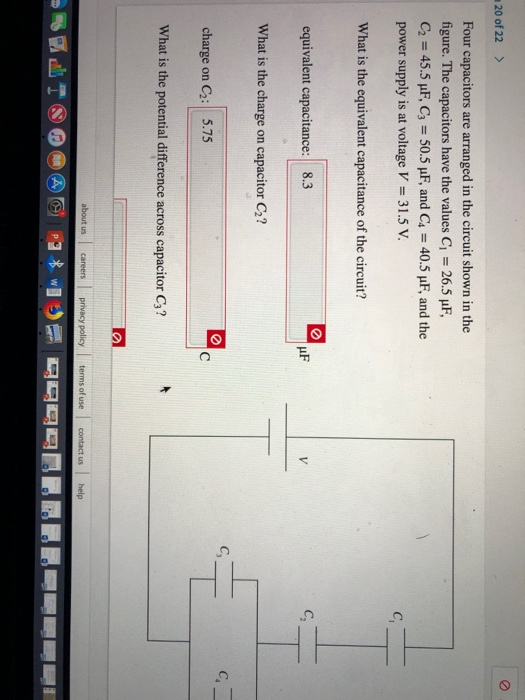20 of 22 > Four capacitors are arranged in the circuit shown in the figure. The capacitors have the values C 26.5 uF, C2 45.5 uF, C3 50.5 pF, and C4 40.5 uF, and the power supply is at voltage V 31.5 V C What is the equivalent capacitance of the circuit? C equivalent capacitance: 8.3 V What is the charge capacitor C2? on C. charge C2: 5.75 C on What is the potential difference across capacitor C3? about us...

• ### Q22 (Capacitors) The figure below shows a 15.0 V battery and four uncharged capacitors of capacitances...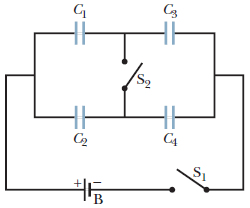Q22 (Capacitors) The figure below shows a 15.0 V battery and four uncharged capacitors of capacitances C1 = 8.00 μF, C2 = 4.00 μF, C3 = 6.00 μF, and C4 = 3.00 μF. If only switch S1 is closed, what is the charge on each capacitor? (a)    C1 __________ μC (b)    C2 __________ μC (c)    C3 __________μC (d)    C4 __________ μC If both switches are closed, what is the charge on each capacitor? (e)    C1 __________μC (f )    C2 __________μC (g)    C3 __________ μC (h)    C4 __________ μC...

• ### Two parallel-plate capacitors are connected in series to a battery as shown in Fig. 24-4. A...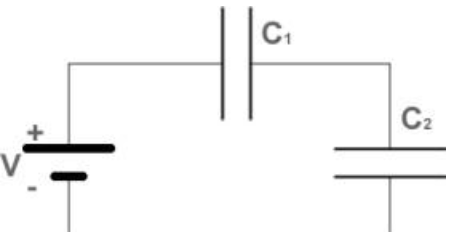Two parallel-plate capacitors are connected in series to a battery as shown in Fig. 24-4. A dielectric is inserted in capacitor C1.What happens to the charge on capacitor C2?